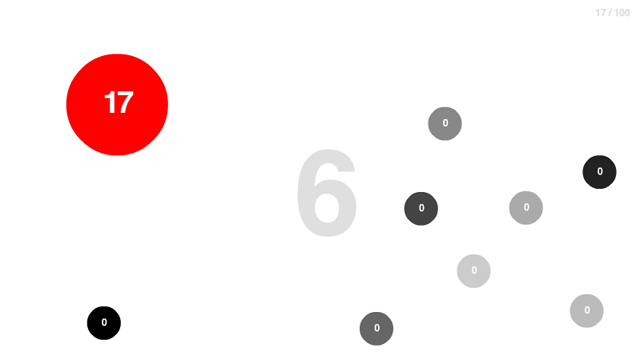# “Hundreds” Flash game prototype using Box2D

Did you play iOS Hundreds game? It’s basically the remake of Hundreds Flash game which wasn’t made with Box2D as far as I can see from the way it handles collisions, so we are going to improve it with the power of Box2D.The aim of the game is simple: make the ball numbers increase to reach a hundred by touching it with the mouse. Do not grow balls when they touch each other.

In this first step, the game features are:

* There are a number of circles moving at a constant speed and colliding each other
* You can make a circle grow by touching it with the mouse

The prototype uses concepts we have already seen in other games, check the Filler Box2D prototype to make Box2D bodies move at a constant speed and the new Way of an Idea prototype to detect bodies under the mouse.

Here is the code:

```package {
import flash.display.Sprite;
import flash.events.Event;
import Box2D.Dynamics.*;
import Box2D.Collision.*;
import Box2D.Collision.Shapes.*;
import Box2D.Common.Math.*;
public class Main extends Sprite {
private var world:b2World=new b2World(new b2Vec2(0,0),true);
private var worldScale:Number=30;
// speed of circles
private var circleSpeed:Number=5;
public function Main() {
debugDraw();
// adding 10 random circles
for (var i:Number=1; i<=10; i++) {
}
// adding boundary walls
}
private function debugDraw():void {
var debugDraw:b2DebugDraw=new b2DebugDraw();
var debugSprite:Sprite=new Sprite();
debugDraw.SetSprite(debugSprite);
debugDraw.SetDrawScale(worldScale);
debugDraw.SetFlags(b2DebugDraw.e_shapeBit|b2DebugDraw.e_jointBit);
debugDraw.SetFillAlpha(0.5);
world.SetDebugDraw(debugDraw);
}
private function addCircle():void {
var bodyDef:b2BodyDef= new b2BodyDef();
bodyDef.type=b2Body.b2_dynamicBody;
bodyDef.position.Set(Math.round(Math.random()*600+20)/worldScale,Math.round(Math.random()*440+20)/worldScale);
bodyDef.userData={name:"sphere"};
var circleShape:b2CircleShape = new b2CircleShape(10/worldScale);
var fixtureDef:b2FixtureDef = new b2FixtureDef();
fixtureDef.density=1;
fixtureDef.friction=0;
fixtureDef.restitution=0.5;
fixtureDef.shape=circleShape;
var body:b2Body=world.CreateBody(bodyDef);
body.CreateFixture(fixtureDef);
// giving the circle a random direction
var randomAngle:Number=Math.random()*2*Math.PI;
body.SetLinearVelocity(new b2Vec2(circleSpeed*Math.cos(randomAngle),circleSpeed*Math.sin(randomAngle)));
}
private function addWall(pX:Number,pY:Number,w:Number,h:Number):void {
var bodyDef:b2BodyDef=new b2BodyDef();
bodyDef.position.Set(pX/worldScale,pY/worldScale);
var polygonShape:b2PolygonShape=new b2PolygonShape();
polygonShape.SetAsBox(w/2/worldScale,h/2/worldScale);
var fixtureDef:b2FixtureDef=new b2FixtureDef();
fixtureDef.shape=polygonShape;
fixtureDef.density=1;
fixtureDef.restitution=0.5;
fixtureDef.friction=0;
var theWall:b2Body=world.CreateBody(bodyDef);
theWall.CreateFixture(fixtureDef);
}
// the core of the script, looking for the body touched and
// increasing the size of the circle shape
private function queryCallback(fixture:b2Fixture):Boolean {
var touchedBody:b2Body=fixture.GetBody();
if (touchedBody.GetUserData()!=null) {
var fixture:b2Fixture=touchedBody.GetFixtureList();
var circleDef:b2CircleShape=fixture.GetShape() as b2CircleShape;
// setting a max radius of 50 pixels for gameplay purpose
}
}
return false;
}
private function update(e:Event):void {
world.Step(1/30,10,10);
world.ClearForces();
for (var b:b2Body=world.GetBodyList(); b; b=b.GetNext()) {
if (b.GetUserData()!=null) {
// keep moving circles at a constant speed
var velocity:b2Vec2=b.GetLinearVelocity();
var speed:Number=velocity.Length();
var ratio:Number=circleSpeed/speed;
velocity.Multiply(ratio);
b.SetLinearVelocity(velocity);
}
}
// checking for bodies under the mouse
world.QueryPoint(queryCallback,new b2Vec2(mouseX/worldScale,mouseY/worldScale));
world.DrawDebugData();
}
}
}```

In this prototype there is a limit to circle growth because I still have to check for collisions and I don't want circles to get bigger than the screen. This is the result:

Move the mouse over a circle to make it grow.

Next time, the complete prototype with the count to 100 and the collision management.

214 GAME PROTOTYPES EXPLAINED WITH SOURCE CODE
// 1+2=3
// 10000000
// 2 Cars
// 2048
// Avoider
// Ballz
// Block it
// Blockage
// Bloons
// Boids
// Bombuzal
// Breakout
// Bricks
// Columns
// CubesOut
// Dots
// DROP'd
// Dudeski
// Eskiv
// Filler
// Fling
// Globe
// HookPod
// Hundreds
// InkTd
// Iromeku
// Lumines
// Magick
// MagOrMin
// Maze
// Memdot
// Nano War
// Nodes
// o:anquan
// Ononmin
// Pacco
// Phyballs
// Platform
// Poker
// Pool
// Poux
// Pudi
// qomp
// Racing
// Renju
// SameGame
// Security
// Sling
// Slingy
// Sokoban
// Splitter
// Sproing
// Stack
// Stringy
// Sudoku
// Tetris
// Threes
// Toony
// Turn
// TwinSpin
// vvvvvv
// Wordle
// Worms
// Yanga
// Zhed
// zNumbers